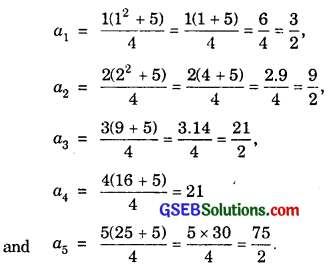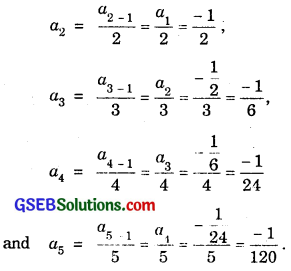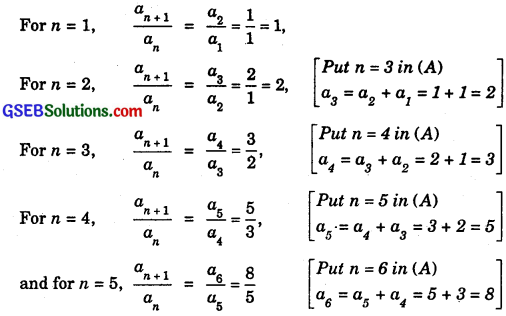# GSEB Solutions Class 11 Maths Chapter 9 Sequences and Series Ex 9.1

Gujarat Board GSEB Textbook Solutions Class 11 Maths Chapter 9 Sequences and Series Ex 9.1 Textbook Questions and Answers.

## Gujarat Board Textbook Solutions Class 11 Maths Chapter 9 Sequences and Series Ex 9.1

Write the first five terms of each of the sequences of questions 1 to 6 whose nth terms are:
Solutions to questions 1 – 6:
1. an = n(n + 2)
2. an = $$\frac{n}{n+1}$$
3. an = 2n
4. an = $$\frac{2n-3}{6}$$
5. an = (- 1)n-15n+1
6. an = $$\frac{n\left(n^{2}+5\right)}{4}$$
Solution to questions 1 – 6:
1. an = n(n + 2)
Putting n = 1, 2, 3, 4 and 5, we get
a1 = 1(1 + 2) = 1.3 = 3, a2 = 2.(2 + 2) = 2.4 = 8,
a3 = 3(3 + 2) = 3.5= 15, a4 = 4.(4 + 2) = 4.6 = 24 and
a5 = 5(5 + 2) = 5.7 = 35.
∴ The first of five terms of the sequence, whose nth term is
an = n(n + 2) are 3, 8, 15, 24 and 35.2. an = $$\frac{n}{n+1}$$
Putting n = 1, 2, 3, 4 and 5, we get
a1 = $$\frac{1}{1+1}$$ = $$\frac{1}{2}$$, a2 = $$\frac{2}{2+1}$$ = $$\frac{2}{3}$$,
a3 = $$\frac{3}{3+1}$$ = $$\frac{3}{4}$$, a4 = $$\frac{1}{4+1}$$ = $$\frac{4}{5}$$
and a5 = $$\frac{5}{5+1}$$ = $$\frac{5}{6}$$.
∴ The first five terms of the sequence whose
nth term is $$\frac{n}{n+1}$$ are $$\frac{1}{2}$$, $$\frac{2}{3}$$, $$\frac{3}{4}$$, $$\frac{4}{5}$$ and $$\frac{5}{6}$$.

3. an = 2n. Putting n = 1, 2, 3, 4 and 5, we get
a1 = 21 = 2, a2 = 4, a3 = 23 = 8,
a4 = 24 = 16 and a5 = 25 = 32.
∴ Required first five terms of the sequence are 2, 4, 8, 16 and 32.4. Here, an = $$\frac{2n-3}{6}$$.
Putting n = 1, 2, 3, 4 and 5, we get
a1 = $$\frac{2×1-3}{6}$$ = $$\frac{2-3}{6}$$ = $$\frac{-1}{6}$$;
a2 = $$\frac{2×2-3}{6}$$ = $$\frac{4-3}{6}$$ = $$\frac{1}{6}$$;
a3 = $$\frac{2×3-3}{6}$$ = $$\frac{6-3}{6}$$ = $$\frac{3}{6}$$ = $$\frac{1}{2}$$;
a4 = $$\frac{2×4-3}{6}$$ = $$\frac{8-3}{6}$$ = $$\frac{5}{6}$$
and a5 = $$\frac{2×5-3}{6}$$ = $$\frac{10-3}{6}$$ = $$\frac{7}{6}$$.

5. an = (- 1)n-15n+1
a1 = (- 1)0.5i+1 = 52 = 25,
a2 = (- 1)2-1.52+1 = – 53 = – 125,
a3 = (- 1)3-1.53+1 = 51 = 625,
a1 = (- 1)4-1.54+1 = – 55 = – 3125,
and a5 = (- 1)5-1.55+1 = 56 = 15625.
The first five terms of the given sequence are
25, – 125, 625, – 3125 and 15625.

6. an = $$\frac{n\left(n^{2}+5\right)}{4}$$
Putting n = 1, 2, 3, 4 and 5, we get∴ The first five terms are $$\frac{3}{2}$$, $$\frac{9}{2}$$ $$\frac{21}{2}$$, 21 and $$\frac{75}{2}$$.Find the indicated terms in each of the following sequences in questions 7 to 10 whose nth terms are
7. an = 4n – 3; a17, a24
8. an = $$\frac{n^{2}}{2^{n}}$$; a7
9. an = (- 1)n-1n3, a9
10. $$\frac{n(n-2)}{n+3}$$; a20
Solutions to questions 7 to 10:
7. an = 4n – 3, Putting n = 17 and 24, we get
a17 = 4.17 – 3 = 68 – 3 = 65
and a24 = 4.24 – 3 = 96 – 3 = 93.

8. an = $$\frac{n^{2}}{2^{n}}$$; a7 Putting n = 7, we get
a7 = $$\frac{7^{2}}{2^{7}}$$ = $$\frac{49}{128}$$.

9. an = (- 1)n-1n3, Putting n = 9, we get
an = (- 1)9-193 = 729.10. an = $$\frac{n(n-2)}{n+3}$$. Putting n = 20, we get
a20 = $$\frac{20(20-2)}{20+3}$$ = $$\frac{20.18}{23}$$ = $$\frac{360}{23}$$.

Write the first five terms of each of the sequences in questions 11 to 13 and obtain the corresponding series:
11. a1 = 3, an = 3an-1 + 2 for all n > 1.
12. a1 = – 1, an = $$\frac{a_{n-1}}{n}$$, n ≥ 2
13. a1 = a2 = 2, an = an-1 – 1, n ≥ 2
Solution to questions 11 to 13:
11. a1 = 3, an = 3an-1 + 2.
Putting n = 2, 3, 4 and 5, we get
a2 = 3a1 + 2 = 3.3 + 2 = 9 + 2 = 11,
a3 = 3a2 + 2 = 3.11 + 2 = 33 + 2 = 35,
a4 = 3a3 + 2 = 3.35 + 2 = 105 + 2 = 107.
and a5 = 3a4 + 2 = 3.107 + 2 = 321 + 2 = 323.
The first five terms of the sequence are 3, 11, 35, 107 and 323.
∴ The corresponding series is 3 + 11 + 35 + 107 + 323 + ……………..12. Here, a1 = – 1, an = $$\frac{a_{n-1}}{n}$$, n ≥ 2.
Putting n = 2, 3, 4, 5 and 6, we get∴ The first five terms of the sequence are – 1, – $$\frac{1}{2}$$, – $$\frac{1}{6}$$, – $$\frac{1}{24}$$ and $$\frac{- 1}{120}$$.
The corresponding series is – 1, – $$\frac{1}{2}$$ – $$\frac{1}{6}$$ – $$\frac{1}{24}$$ – $$\frac{1}{120}$$ – …………………

13. Here, a1 = a2 = 2, an = an-1 – 1 (n > 2)
Putting n = 3, 4, 5, 6 and 7, we get
a3 = a3-1 – 1 = a2 – 1 = 2 – 1 = 1;
a4 = a4-1 – 1 = a3 – 1 = 1 – 1 = 0;
a5 = a5-1 – 1 = a4 – 1 = 0 – 1 = – 1;
The first five terms of the sequences are 2, 2, 1, 0 and – 1.
The corresponding series is 2 + 2 + 1 + 0 – 1 + …………………14. The fibonacci sequence is defined by 1 = a1 = a2 and an = an-1 + an-2, n > 2.
Find $$\frac{a_{n+1}}{a_{n}}$$ for n = 1, 2, 3, 4 and 5.
Solution:
Here, a1 = 1 = a2 and an = an-1 + an-2 (n > 2) …………….. (A)The values of $$\frac{a_{n+1}}{a_{n}}$$ for n = 1, 2, 3, 4 and 5 are respectively 1, 2, $$\frac{3}{2}$$, $$\frac{5}{3}$$ and $$\frac{8}{5}$$.